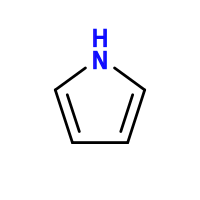# pyrrole¶

A Python package for solving chemical problems with computational modeling.

## Usage example¶

As a usage example, let’s calculate the energy barrier involved in nitrogen inversion in ammonia.When ammonia turns “inside out”, it passes through a planar transition state (image in public domain).

We do this in three simple steps (only eight lines of code):

1. Get the data

We first obtain the raw data, which will later be fed to our chemical model. Below we read computational chemistry logfiles of both ground and transition states .

>>> from pyrrole.atoms import read_cclib, create_data
>>> data = create_data(gs, ts)


Pyrrole uses cclib for reading logfiles, which is compatible with all major computational chemistry packages. You could also want to read tabular data from a file (or even from the web) using pandas.

1. Specify the model

We now describe our model. This is accomplished through chemical equations:

>>> from pyrrole import ChemicalEquation
>>> equation = ChemicalEquation("NH3(g) -> NH3(g)#", data)


While model above consists of a single ChemicalEquation, you could create complex models with multiple chemical equations with ChemicalSystem objects. You might want to store your complex models in text files too.

1. Obtain the results

Simply let pyrrole calculate the energy barrier:

>>> results = equation.to_series()
>>> results["freeenergy"] * 2625.4996382852164  # Hartree to kJ/mol
19.30952589472923


(As a side note, the reference value is 21.162 kJ/mol .)

Interested? Have another example.

  Optimizations and frequency calculations of both ammonia and the planar transition state were performed at PBEh-3c using the ORCA electronic structure package (version 4.0.1.2). Logfiles can be found in the project’s repository.
  Chem. Phys. Lett., 2003, 370 (3), pp 360-365 DOI: 10.1016/S0009-2614(03)00107-6.

## Installation¶

You can get the library directly from PyPI:

\$ pip install pyrrole